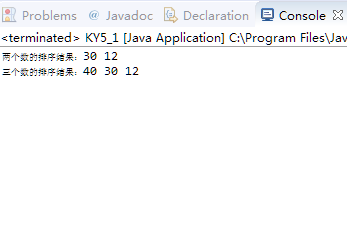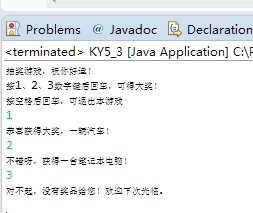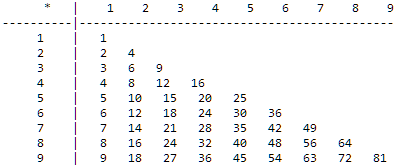java实验（五）

Windows7系统平台下的eclipse

（一）类的多态性练习

class IntSort {
public String sort (int a, int b) {
if (a>b)
return a+" "+b;
else
return b+" "+a;
}
public String sort (int a, int b, int c) {
int swap;
if (a<b) {
swap=a;
a=b;
b=swap;
}
if (a<c) {
swap=a;
a=c;
c=swap;
}
if (b<c) {
swap=b;
b=c;
c=swap;
}
return a+" "+b+" "+c;
}
}

public class KY5_1 {
public static void main(String args[])
{
int a=30, b=12, c=40;
IntSort s=new IntSort();
System.out.println("两个数的排序结果："+s.sort(a,b));
System.out.println("三个数的排序结果："+s.sort(a,b,c));
}
}


（4）程序的输出的结果：（5）

I.public String sort (int a, int b) 这个是输出a,b两个数的值。

II.public String sort (int a, int b, int c)调用第二个方法中的对比对比大小，按照从大到小的功能输出。

（6）

调用了方法I：s.Sort(a, b)。调用方法II：s.Sort(a, b，c)

（二）构造方法的重载

class RunDemo {
RunDemo(String name) {
}
RunDemo(String name, String pwd) {
this(name);
}
void check() {   //检验用户名和密码
String s=null;
else
s="用户名不能为空！";
s=s+" 口令无效！";
else
s=s+" 口令：******";
System.out.println(s);
}
}

public class KY5_2 {
public static void main(String[] args) {
RunDemo r1=new RunDemo("刘新宇");
r1.check();
RunDemo r2=new RunDemo(null, "654321");
r2.check();
RunDemo r3=new RunDemo("张驰","123456");
r3.check();
}
}


（3）用户名：刘新宇 口令无效！

（4）

I.new RunDemo("刘新宇");调用了“RunDemo(String name)” 的构造方法。

II.new RunDemo(null, "654321");“xiaowu.RunDemo.RunDemo(String, String)”的构造方法。

III.new RunDemo("张驰","12345678");“xiaowu.RunDemo.check()”的构造方法。

（三）使用键盘输入

    import java.io.*;
class KY5_3 {
public static void main(String args[]) throws IOException {
char ch;
System.out.println("抽奖游戏，祝您好运!");
System.out.println("按 1、2、3 数字键后回车，可得大奖!");
System.out.println("按空格键后回车，可退出本游戏.");
{
System.in.skip(2);     // 跳过回车键
switch (ch) {
case '1':
System.out.println("恭喜您得大奖，一辆汽车!");
break;
case '2':
System.out.println("不错呀，您得到一台笔记本电脑!");
break;
case '3':
System.out.println("没有白来，您得到一台冰箱!");
break;
default:
System.out.println("对不起，没有奖品给您!欢迎下次光临。");
}
}
}
}


4.（四）使用多重循环语句

public class KY5_4
{
public static void main(String args[])
{
int i, j, n=9;
System.out.print("      *   |");
for (i=1; i<=n; i++)  { System.out.print("    "+i);  }   //第一个for循环
System.out.print("\n----------|");
for (i=1; i<=n; i++)  { System.out.print("-----");  }     //第二个for循环
System.out.println();
for (i=1; i<=n; i++)                               //第三个for循环
{
System.out.print("     "+i+"    |");
for (j=1; j<=i; j++)  { System.out.print("   "+i*j);  }   //第四个for循环
System.out.println();
}
}
}


4.For循环的作用：

（五）实验总结：